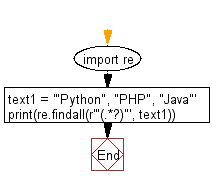﻿ Python Exercises: Extract values between quotation marks of a string - w3resource# Python: Extract values between quotation marks of a string

## Python Regular Expression: Exercise-38 with Solution

Write a Python program to extract values between quotation marks of a string.

Sample Solution:-

Python Code:

``````import re
text1 = '"Python", "PHP", "Java"'
print(re.findall(r'"(.*?)"', text1))
```
```

Sample Output:

```['Python', 'PHP', 'Java']
```

Pictorial Presentation:Flowchart:## Visualize Python code execution:

The following tool visualize what the computer is doing step-by-step as it executes the said program:

Python Code Editor:

Have another way to solve this solution? Contribute your code (and comments) through Disqus.

What is the difficulty level of this exercise?

Test your Python skills with w3resource's quiz

﻿

## Python: Tips of the Day

Converts a string to kebab case

Example:

```from re import sub

def tips_kebab(s):
return sub(
r"(\s|_|-)+","-",
sub(
r"[A-Z]{2,}(?=[A-Z][a-z]+[0-9]*|\b)|[A-Z]?[a-z]+[0-9]*|[A-Z]|[0-9]+",
lambda mo: mo.group(0).lower(), s))

print(tips_kebab('sentenceCase'))
print(tips_kebab('Python Tutorial'))
print(tips_kebab('the-quick_brown Fox jumps_over-the-lazy Dog'))
print(tips_kebab('hello-world'))
```

Output:

```sentencecase
python-tutorial
the-quick-brown-fox-jumps-over-the-lazy-dog
hello-world
```